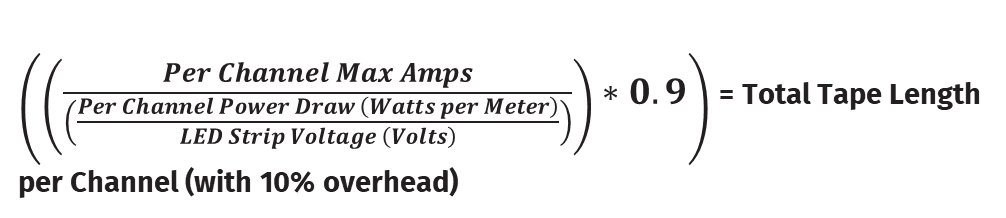It's possible to run multiple CV strips in parallel from a CVC4  (all strips will behave identically).

The CVC4 is rated at a maximum of 5Amps per channel. To work out the maximum amount of tape which can be run from a CVC4, we will need to do the following calculation:Take the channel with the highest power draw (normally Red in RGB and White in RGBW); in this case, it is 4.45W/meter. Divide that by 24v (the voltage of the CV strip), which is 0.18. This is then divided by 5 (maximum Amps per Channel). Before multiplying by 0.9 to provide us with the 10% overhead. This calculates that our maximum run is 25m.That 25m is the maximum length that can be run from a CVC4 in a single line. If you wish to run multiple CV strips in parallel, then this 25m would be the maximum length of the runs added together ( for example, 2*12.5m / 3*8m).

To add extra levels of protection to your installation, it is recommended that an appropriate fuse is added to the VCC line between the CVC4 and each VCC line on the CV strip. To determine the appropriate fuse:

- Add the maximum power draw per meter (in Watts)

- Multiply that by the total length of the strip to give the total power draw in Watts.

- Next, divide that by the voltage of the strip.

- Multiply this final value by 1.1 to give a 10% overhead.

- Finally, round this up to the closest available fuse current rating.

Additionally, the CVC4 has a 20Amp fuse built into the device; this can be replaced or downgraded to match the project's requirements. If you have multiple strips with a current draw is significantly lower than 20Amp blade fuse in the CVC4, it's recommended you replace it with a fuse with a lower current rating to give protection better suited to your setup.

Note: never fit a fuse that exceeds the CVC4's 20A current capacity